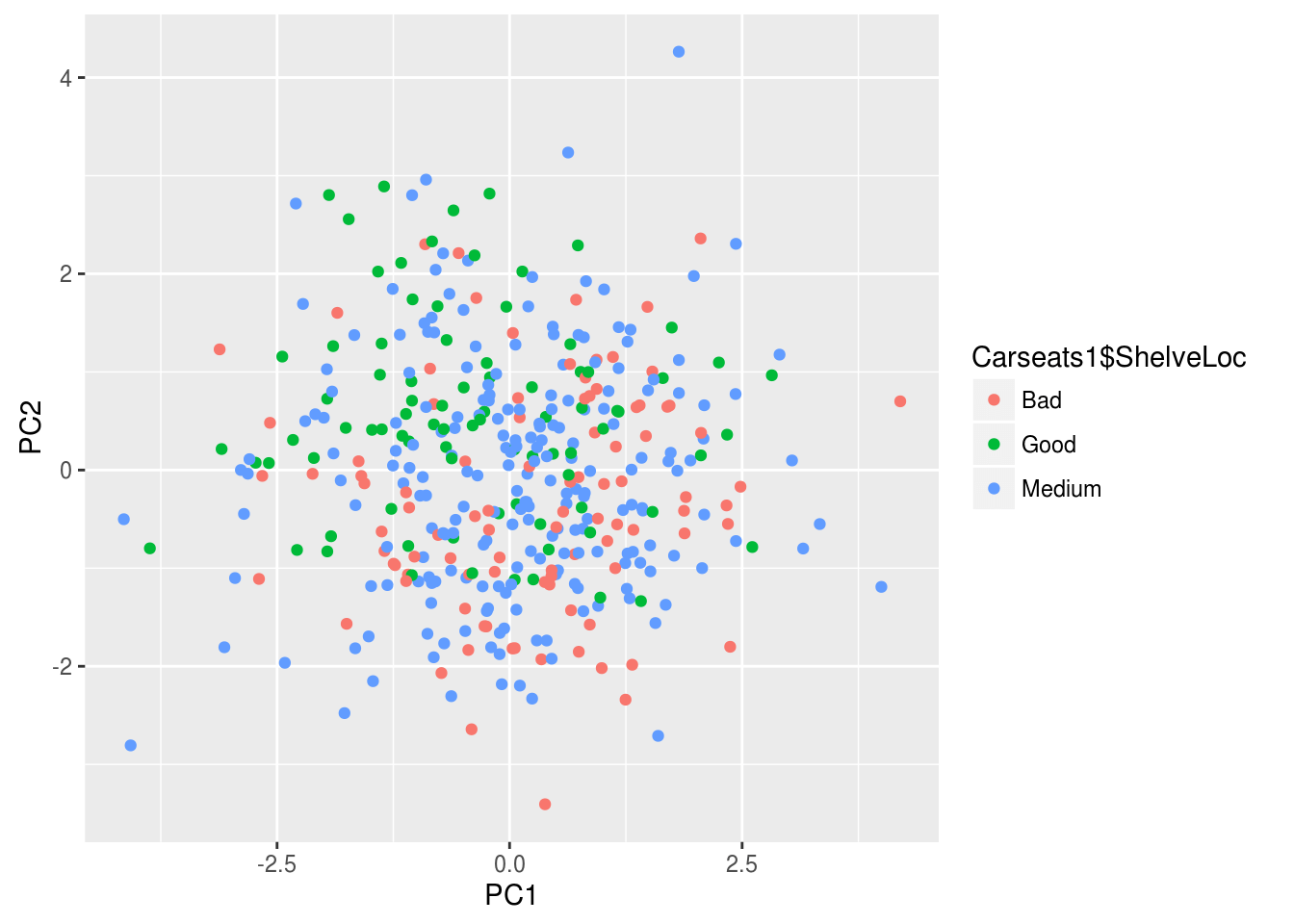# Principal Component Analysis in R

This post will demonstrate the use of principal component analysis (PCA). PCA is useful for several reasons. One it allows you place your examples into groups similar to linear discriminant analysis but you do not need to know beforehand what the groups are. Second, PCA is used for the purpose of dimension reduction. For example, if you have 50 variables PCA can allow you to reduce this while retaining a certain threshold of variance. If you are working with a large dataset this can greatly reduce the computational time and general complexity of your models.

Keep in mind that there really is not a dependent variable as this is unsupervised learning. What you are trying to see is how different examples canbe mapped in space based on whatever independent variables are used. For our example, we will use the “Carseats” dataset from the “ISLR”. Our goal is to understand the relationship among the variables when examining the shelve location of the car seat. Below is the initial code to begin the analysis

``````library(ggplot2)
library(ISLR)
data("Carseats")``````

We first need to rearrange the data and remove the variables we are not going to use in the analysis. Below is the code.

``````Carseats1<-Carseats
Carseats1<-Carseats1[,c(1,2,3,4,5,6,8,9,7,10,11)]
Carseats1\$Urban<-NULL
Carseats1\$US<-NULL``````

Here is what we did 1. We made a copy of the “Carseats” data called “Careseats1” 2. We rearranged the order of the variables so that the factor variables are at the end. This will make sense later 3.We removed the “Urban” and “US” variables from the table as they will not be a part of our analysis

We will now do the PCA. We need to scale and center our data otherwise the larger numbers will have a much stronger influence on the results than smaller numbers. Fortunately, the “prcomp” function has a “scale” and a “center” argument. We will also use only the first 7 columns for the analysis as “sheveLoc” is not useful for this analysis. If we hadn’t moved “shelveLoc” to the end of the dataframe it would cause some headache. Below is the code.

``````Carseats.pca<-prcomp(Carseats1[,1:7],scale. = T,center = T)
summary(Carseats.pca)``````
``````## Importance of components:
##                           PC1    PC2    PC3    PC4    PC5     PC6     PC7
## Standard deviation     1.3315 1.1907 1.0743 0.9893 0.9260 0.80506 0.41320
## Proportion of Variance 0.2533 0.2026 0.1649 0.1398 0.1225 0.09259 0.02439
## Cumulative Proportion  0.2533 0.4558 0.6207 0.7605 0.8830 0.97561 1.00000``````

The summary of “Carseats.pca” Tells us how much of the variance each component explains. Keep in mind that the number of components is equal to the number of variables. The “proportion of variance” tells us the contribution each component makes and the “cumulative proportion”.

If your goal is dimension reduction than the number of components to keep depends on the threshold you set. For example, if you need around 90% of the variance you would keep the first 5 components. If you need 95% or more of the variance you would keep the first six. To actually use the components you would take the “Carseats.pca\$x” data and move it to your data frame.

Keep in mind that the actual components have no conceptual meaning but is a numerical representation of a combination of several variables that were reduced using PCA to fewer variables such as going from 7 variables to 5 variables.

This means that PCA is great for reducing variables for prediction purpose but is much harder for explanatory studies unless you can explain what the new components represent.

For our purposes, we will keep 5 components. This means that we have reduced our dimensions from 7 to 5 while still keeping almost 90% of the variance. Graphing our results is tricky because we have 5 dimensions but the human mind can only conceptualize 3 at the best and normally 2. As such we will plot the first two components and label them by shelf location using ggplot2. Below is the code

``````scores<-as.data.frame(Carseats.pca\$x)
pcaplot<-ggplot(scores,(aes(PC1,PC2,color=Carseats1\$ShelveLoc)))+geom_point()
pcaplot``````From the plot, you can see there is little separation when using the first two components of the PCA analysis. This makes sense as we can only graph to components so we are missing a lot of the variance. However, for demonstration purposes the analysis is complete.